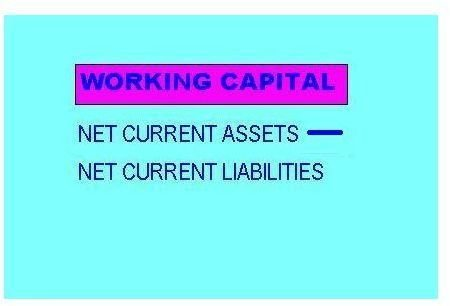# Learn How to Calculate Working Capital

Working capital is the difference between the current assets or short term assets that a company holds and the current liabilities orthe short term liabilities which the company has to dispose of within the operating cycle under consideration.

The formula to calculate working capital is:

Working Capital = Net Current Assets – Net Current Liabilities

Current assets or short-term assets are assets that the company either uses or disposes within one operating cycle, usually a year. Examples include cash or cash equivalents, current inventory, accounts receivable from sale of goods, and marketable securities. In contrast, long-term assets such as land and machinery are of a more permanent nature.

Current liabilities or short-term liabilities are current debt obligations, accrued expenses, notes payable, portions of long-term debt classified as current, and other accounts payables that a company has to fulfill any time soon, within one operating cycle, usually a year. This is in contrast to long-term liabilities such as long-term debt. Accounting standards dictate that long-term debt remains excluded from current liabilities even if such debts expire within the operational cycle.

The working capital formula to calculate net working capital per dollar of sales is:

Working Capital per dollar of sales = Working Capital / Total Sales

The value for total sales comes from the company’s income statement

## Illustrative Example

Assume a company has the following values derived from the financial statements:

• Cash on hand: \$100,000
• Accounts receivable: \$25,000
• Securities: \$120,000
• Inventory: \$50,000
• Value of Machinery (after depreciation): \$25,000
• Value of Land: \$20,000
• Accounts payable: \$85,000
• Current debt: \$40,000
• Accrued expenses: \$35,000
• Total sales: \$650,000

The first step to calculate working capital is identifying current assets. Here, the current assets are cash on hand, accounts receivables, securities, and inventory.

Current Assets = \$100,000 + \$25,000 + \$120,000 + \$50,000 = \$ 295,000.

The next step is calculating current liabilities. In the given examples, account payables, current debt, and accrued expenses are current liabilities.Current Liabilities = \$85,000 + \$40,000 + \$35,000 = \$160,000

The final step is to calculate the working capital.

Working Capital = Current Assets – Current Liability = \$295,000 – \$160,000 = \$135,000

To identify the working capital per dollar of sales, divide working capital by total sales = \$ 135,000/ \$ 650,000 = 0.207 or 20.7 percent.

There is no optimal working capital ratio, and the ideal level depends on factors such as company’s cash requirement, working capital maintained by industry peers, management strategy, and other factors.

## Distorters

The definition of current assets can distort the calculations of the working capital ratio. The allocation of assets into short term or current assets, and long term or fixed assets are very often ambiguous and contextual. A general guideline is to include disposable assets quickly, within the operational cycle as current assets, but what items make the cut depends on managerial discretion.

Many companies underestimate the importance of working capital. Lack of adequate working capital can lead to severe cash crunches for a company, leaving it with no money to fund their day-to-day operations such as payment of salaries, settlement of bills, and the like. Working capital, however, generates very little returns and as such, some companies try to devise ways to manage their operations while working around working capital requirements. Concepts such as zero working capital are steps in this direction.

## Reference

1. Wikipedia: "Working Capital." Retrieved from https://en.wikipedia.org/wiki/Working_capital on 12 February 2011.
2. Investopedia: "Working Capital." Retrieved from https://www.investopedia.com/terms/w/workingcapital.asp on 12 February 2011.

Image by N Nayab